# 两边夹的应用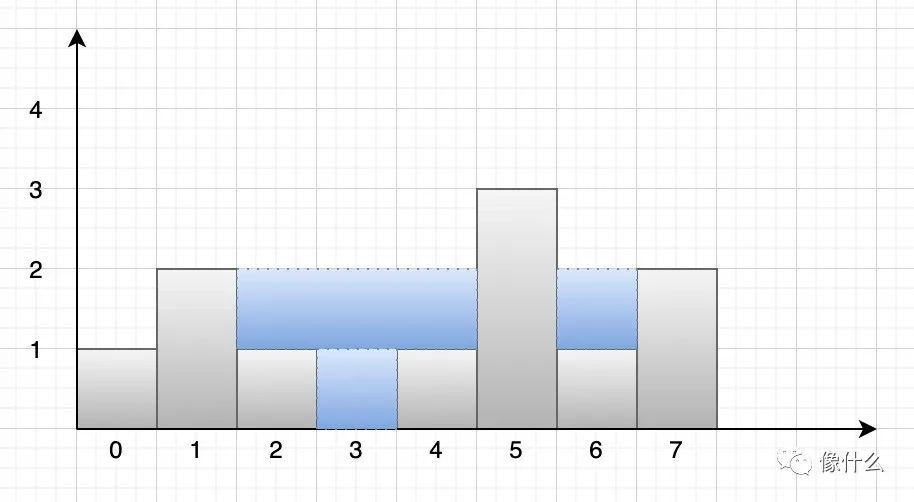﻿

﻿

﻿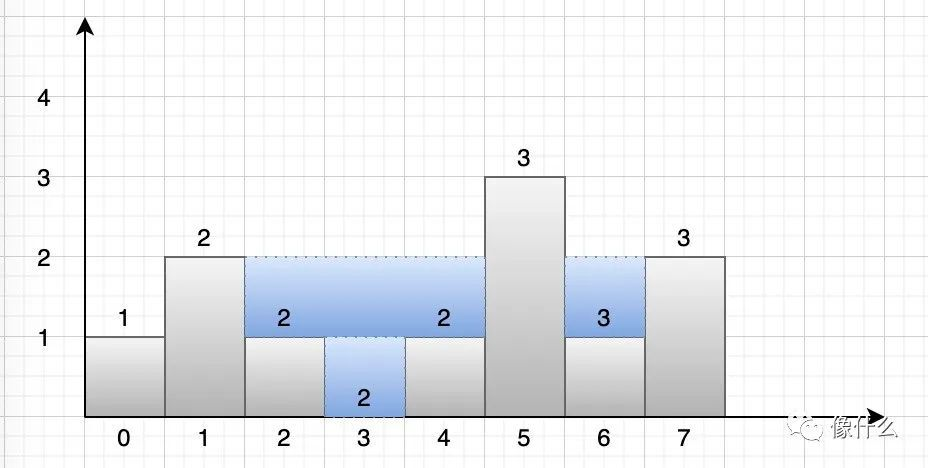﻿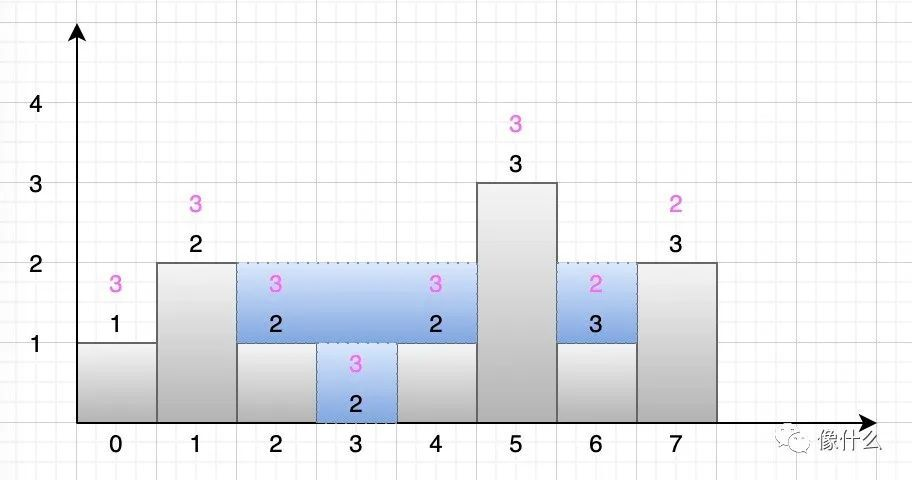﻿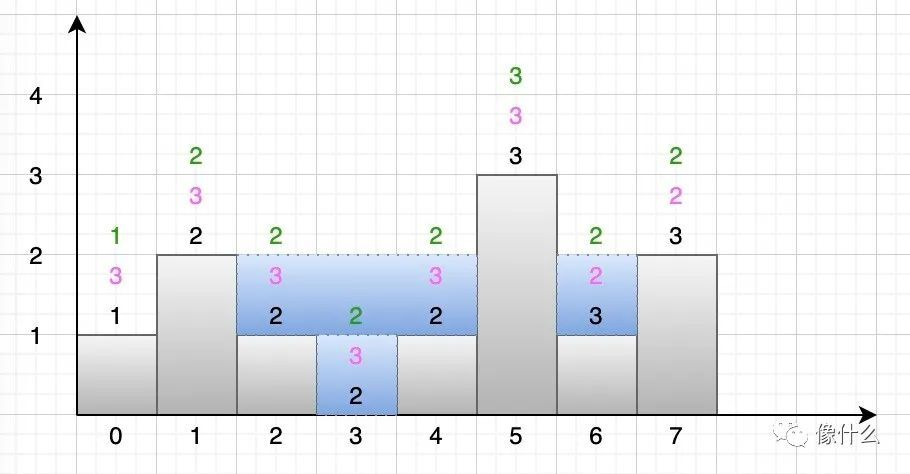﻿

public int trap(int[] height) {    int length = height.length;    if (height == null || length < 3) {        return 0;    }    int[] maxLeft = new int[length];    int[] maxRight = new int[length];    for (int i = 0; i < length; i++) {        if (i == 0) {            maxLeft = height;            maxRight[length - 1] = height[length - 1];        } else {            maxLeft[i] = Math.max(maxLeft[i - 1], height[i]);            maxRight[length - 1 - i] = Math.max(maxRight[length - i], height[length - 1 - i]);        }    }    int total = 0;    for (int i = 0; i < length; i++) {        total += Math.min(maxLeft[i], maxRight[i]) - height[i];    }    return total;}

﻿

﻿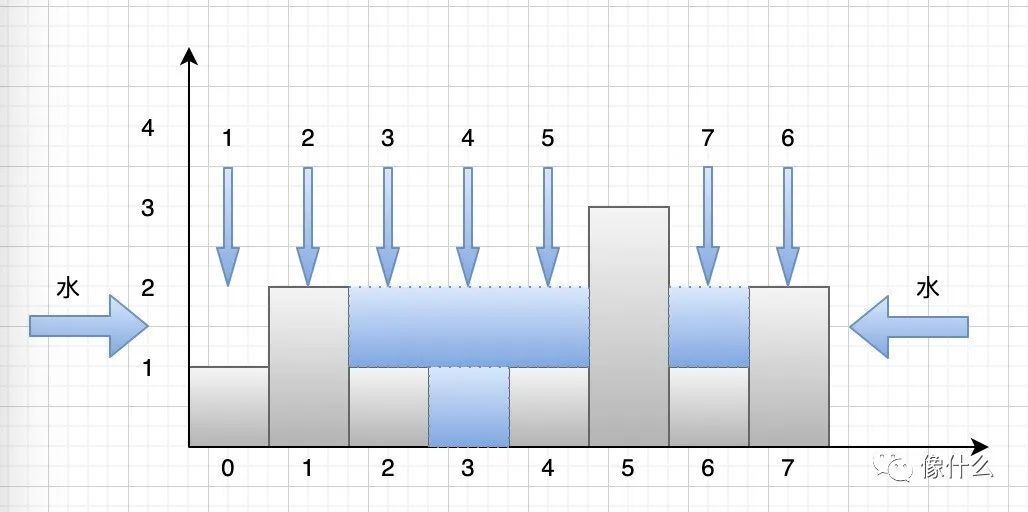﻿

public int trap(int[] height) {    if (height == null || height.length < 3) {        return 0;    }    int total = 0, maxLeft = 0, maxRight = 0;    int leftIndex = 0, rightIndex = height.length - 1;    while (leftIndex < rightIndex) {        if (height[leftIndex] <= height[rightIndex]) {            maxLeft = Math.max(maxLeft, height[leftIndex]);            total += maxLeft - height[leftIndex];            leftIndex++;        } else {            maxRight = Math.max(maxRight, height[rightIndex]);            total += maxRight - height[rightIndex];            rightIndex--;        }    }    return total;}

﻿

for (int leftIndex = 0, rightIndex = height.length - 1; leftIndex < rightIndex; )

﻿## 评论